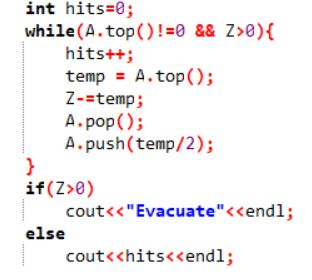# SAVKONO - Editorial

Author: Sandeep Singh
Tester: Arkapravo Ghosh
Editorialist: Sandeep Singh

SIMPLE

Priority Queue

# PROBLEM:

There are N soldiers with power levels A_1, A_2....., A_N, When ith soldier attacks Pain, his strength ( Z ) gets reduced by the corresponding power of the attacking soldier. The power of the attacking soldier also gets halved. What is the minimum no. of attacks required, if at all possible, to defeat Pain.

# QUICK EXPLANATION:

The solution to this problem is a simple implementation of a priority queue. The highest power, P_M, is popped and subtracted from strength Z and [P_M/2] is pushed in the queue. This is repeated until Z reduces to 0 or less or P_M pops out to be 0. The answer is the no. of times the loop runs or “Evacuate” based on the value of Z after the above operation is over.

# EXPLANATION:

To minimize the no. of attacks, the optimum choice would be to attack with the current highest power available. To get the highest power each time in optimal time we insert all the powers in the Priority Queue. After that, we keep popping out the highest power from the Queue returning half of it to the queue till Pain is defeated or no more attacks are possible i.e. all powers are reduced to 0 (Max popped power is 0).
If Z is reduced to 0 or less, this means Pain can be defeated and the minimum no. of attacks required to do so is the no. of times the loop was run or Priority Queue was popped. Else Pain cannot be defeated and “Evacuate” is to be printed.
Working with Priority queue is very simple and self explanatory. Below is a snippet of working of priority queue A.Time complexity is O(N * logN * logA)

# SOLUTIONS:

Setter's Solution
``````
#include <bits/stdc++.h>
using namespace std;

int main() {

//freopen("ip5.in", "r", stdin);
//freopen("op5.out", "w", stdout);

int t ;
cin>>t;
while(t--) {
int N, i,Z,temp;
cin>>N>>Z;
priority_queue<int> A;
for(i=0;i<N;i++){
cin>>temp;
A.push(temp);
}
int hits=0;
while(A.top()!=0 && Z>0){
hits++;
temp = A.top();
Z-=temp;
A.pop();
A.push(temp/2);
}
if(Z>0)
cout<<"Evacuate"<<endl;
else
cout<<hits<<endl;
}
return 0;
}
``````
Tester's Solution
``````    CodeChef submission 26040101 (C++14) plaintext list. Status: AC, problem SAVKONO, contest
ENAU19TS. By horsbug98 (horsbug98), 2019-08-20 22:22:53.
#include <bits/stdc++.h>
using namespace std;

#define ll long long
cin.tie(0); cout.tie(0);
#define T int tt; cin>>tt; while(tt--)

int main() {
fastio;
//find_primes();
//binomial_pre();
//freopen("input.txt", "r", stdin);
//freopen("output.txt", "w", stdout);
T {
int n, z, x, res = 0;
cin >> n >> z;
priority_queue<int> pq;
for(int i = 0; i < n; ++i) {
cin >> x;
pq.push(x);
}
while(!pq.empty()) {
if(z <= 0)
break;
x = pq.top(); pq.pop();
z -= x;
x >>= 1;
if(x) pq.push(x);
++res;
}
if(z > 0)
cout << "Evacuate\n";
else
cout << res << '\n';
}
return 0;
}
``````
7 Likes

Nice Editorial. Nice Question. I used priority-queue in a contest for the first time in my life…thanks to this question7 Likes

@anon55659401 really ?Yes, I didn’t see the use of priority queue in any contest in last few months.

My solution .
Used hashing instead of a priority queue.Nice Approach. Thanks for sharing your solution

Please tell me why is this wrong?

#include <bits/stdc++.h>

using namespace std;

int main()
{
int t,buf=0;
cin>>t;

``````while(t--){

int n,pow,count=0;
cin>>n>>pow;

int a[n];
for(int i=0;i<n;i++)
cin>>a[n];

while(pow>0){

sort(a,a+n);

if(a[n]!=0){
pow=pow-a[n];
a[n]=a[n]/2;

count++;
}
else{
buf=1;
cout<<"Evacuate"<<endl;
break;
}
}

if(buf==0)
cout<<count<<endl;
}
``````

}

``````#include <bits/stdc++.h>
using namespace std;

int main()
{
#define int long long int
std::ios_base::sync_with_stdio(false);
cin.tie(NULL);
int t;
cin>>t;
while(t--)
{
int n,z,i,temp,count,l;
cin>>n>>z;
map<int,int,greater<int> > m;
for(i=0;i<n;i++)
{
int x;
cin>>x;
m[x]++;
}
temp=z;
count=0;
map<int,int,greater<int> > :: iterator itr;

while(true)
{

if(m.empty())
{
cout<<"Evacuate"<<endl;
break;
}
itr=m.begin();
if(itr->second<1)
m.erase(itr);
l=itr->first;
l=l/2;
temp=temp-(itr->first);
if(l!=0)
m[l]++;
count++;
itr->second--;
if(itr->second==0)
m.erase(itr);
if(temp<=0)
{
cout<<count<<endl;
break;
}
}
}

return 0;
}
``````

how will you implement the same problem by using set

The solution is really nice, but it can be solved in O(n) time->using idea of counting sort.

can you guys help me, coz i’m getting time limit exceeded error.Please resolve this.

#include <bits/stdc++.h>

using namespace std;

int find_no_times(vector soldier, int Z){

``````int max_ele;

vector<int>::iterator it;//for getting the position of the maximum value

int times=0;

while (1)

{

max_ele = *max_element(soldier.begin(), soldier.end());

Z -= max_ele;

it = find(soldier.begin(), soldier.end(), max_ele);

if (it != soldier.end())

{

soldier[(it - soldier.begin())] = max_ele/2;

}

times++;

if (Z <= 0) {return times;}

if ( std::adjacent_find( soldier.begin(), soldier.end(), std::not_equal_to<>() ) == soldier.end() ) //checking whether the all the soldier's having same strength

{

return 0;//100 means soldier hava to evacuate

}

}
``````

}

int main(int argc, char const *argv[])

{

``````int n;

cin>>n;

int N=0, Z=0;

int val;

int defeat=0;

while (n--)

{

cin>>N>>Z;

vector<int> soldier(N,0);

for (int i = 0; i < N; i++)

{

cin>>val;

soldier.push_back(val);

}

defeat = find_no_times(soldier, Z);

if(defeat == 0){cout<<"Evacuate";}

else cout<<defeat;

}

return 0;
``````

}

Can anyone explain me why am i getting a runtime error in this solution.

https://www.codechef.com/viewsolution/39866858

when i ran it on my compiler it worked

https://www.codechef.com/viewsolution/40203538

I realize the recommendation is to use a priority queue. I could do this in c++ and use its priority queue. However, I am choosing to use c#, which does not have a priority queue. So I am using a brute force approach. All of the test cases that I have been able to think up pass. But when I submit I get NZEC. Could someone help me understand why?

https://www.codechef.com/viewsolution/40966273

Why is this getting TLE?

Really nice problem, that teaches how to use priority queues. Thanks!